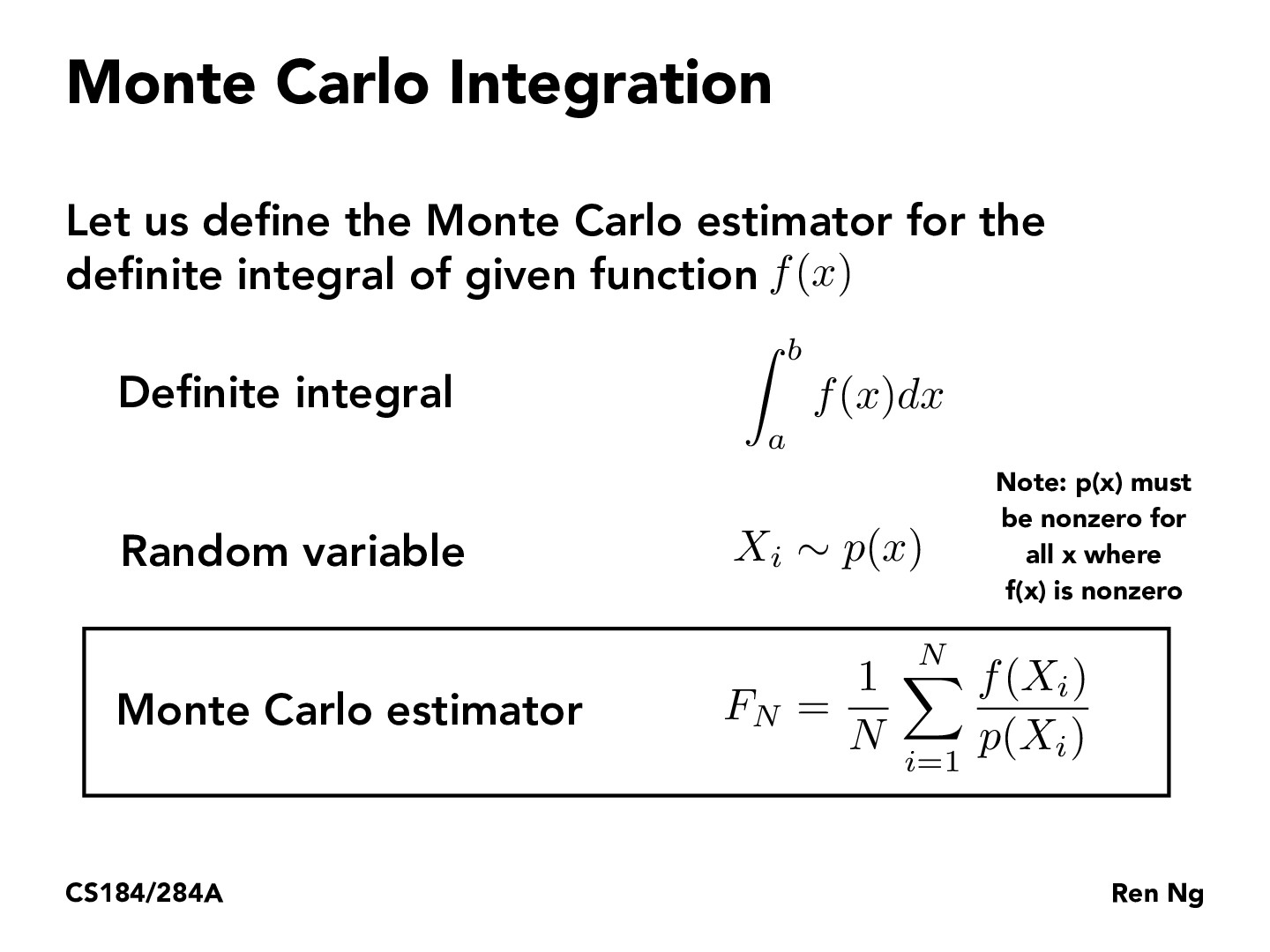Lecture 12: Monte Carlo Integration (21)leonxu1

If I remember correctly, a Monte Carlo algorithm is one that makes use of random samples to compute an answer that is correct on average, but wrong with very small probability. This is in contrast to a Las Vegas algorithm, which also uses randomness and is guaranteed to produce a correct answer. However, a Las Vegas algorithm only has good runtime on average, with a small chance of very bad runtime - one example is quicksort. Monte Carlo on the other hand guarantees a certain runtime even though the accuracy is not guaranteed. It is possible to convert one into the other.

In this case, we estimate lighting using Monte Carlo integration, which gives us an answer that is correct on average, but with a small chance of being very wrong (say if all of the random samples were the same point). If we define "wrong" to be a specific distance from the true value, the probability of being wrong can be made arbitrarily small by taking more sample points.jasonwxu

I find it interesting that we're not scaling f(x_i) by p(x_i) but rather the probability's inverse. This makes intuitive sense as the samples we see frequently (rarely), we would want to down(up)-weight them. Looking at it more analytically, it is interesting (or less intuitive at least to me) that the weightings result in a biased estimator. If the probability for a give sample is .1, the sample is increased 10x while for another sample with probability .8, the sample is increased only by 1.25x.arjunsarup-1

This is a great resource on random variables, variance, Monte Carlo, all that good stuff: http://prob140.org/textbook/Chapter_11/04_Markov_Chain_Monte_Carlo.htmldangeng184

I know that in RL you can choose advantage functions such that the variance of an estimate is minimized. I'm wondering if we can do sort of the same thing here. That is, choose the underlying sampling distribution such that we get less variance. Although what exactly is the optimal sampling distribution seems pretty unclear and seems to depend on a ton of different things, most importantly the shape of f, which I'm not even sure we know fully, in general.dangeng184

WAIT, I just realized what I mentioned above is exactly importance sampling. I looked at the wikipedia page for importance sampling and it seems that there does exist a closed form solution for p in terms of f. But it seems our fundamental problem is that we don't fully know f, or at least not all of it without a crap ton of compute. I like this quote from the wikipedia page: "Choosing or designing a good biased distribution is the "art" of importance sampling." In the example later in this lecture about the rectangle casting a diffuse shadow it really seems that we just lucked out. I think in general you can't just sample rays that pass through the light source, as light can bounce of surfaces. This sounds like sort of a hard problem...jcebreros1

leonxu1 The chance of the Monte Carlo algorithm being wrong goes to 0 as the number of samples grows as shown by the law of large numbers https://en.wikipedia.org/wiki/Law_of_large_numbers#Strong_law

You must be enrolled in the course to comment